## What is a Linked List?

linked list is a linear data structure made of a chain of node objects in which each node object contains a value and a pointer to the next node in the chain.

Linear data Structure: All the data is arranged in linear order• Single linked list- Linked list traversal is in single direction (forward)
• Double linked list – Linked list traversal in both direction (forward and backward)
• Circular linked list – Last element of linked list point to first element of linked list

Linked list is collection is node connected to each other in linear arrangement.

Now Node has two elements

1. Value of that node (or data)
2. Pointer to next item

Last node of linked list refers to null reference. This indicates we have reached the end point of linked list.

Code for creating a node in linked list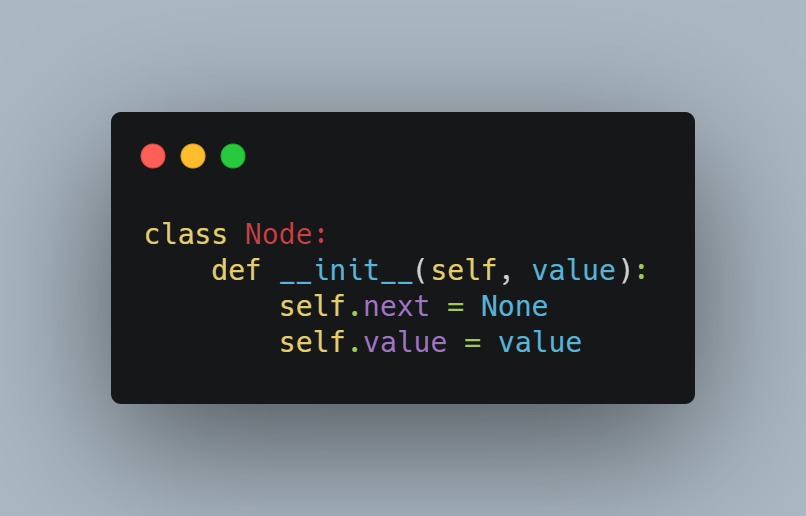Creating a linked list means initializing head node in the linked list. Since linked list is empty, head node is currently pointing to end node ie null reference.Figure: Code for initializing a linked list

Inserting a new node in linked list

We can add new nodes in our linked list. In the below example, we are adding new node with a given value at end of list.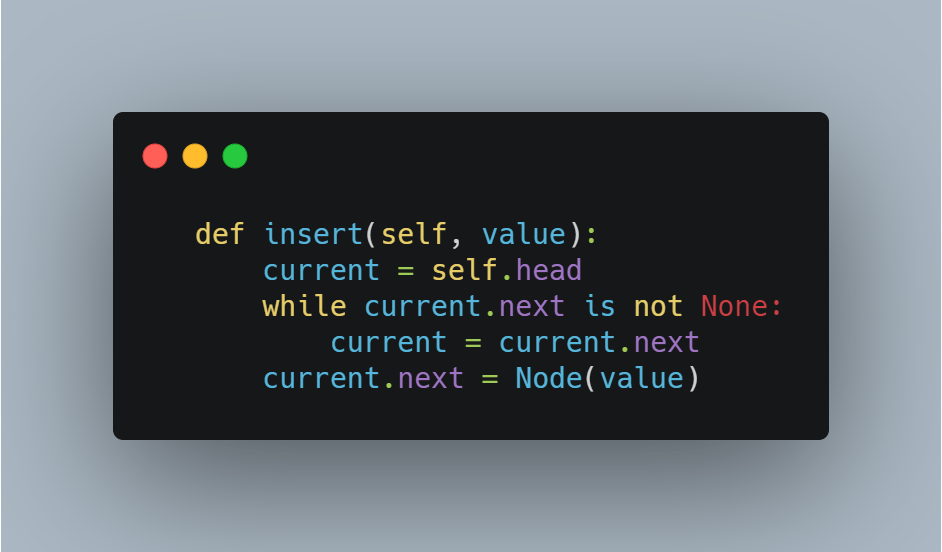Figure: Code for inserting a node in a linked list

Deleting a node

We can delete a given node in the list. In the example shown below, we are deleting a node with a given value in the linked list.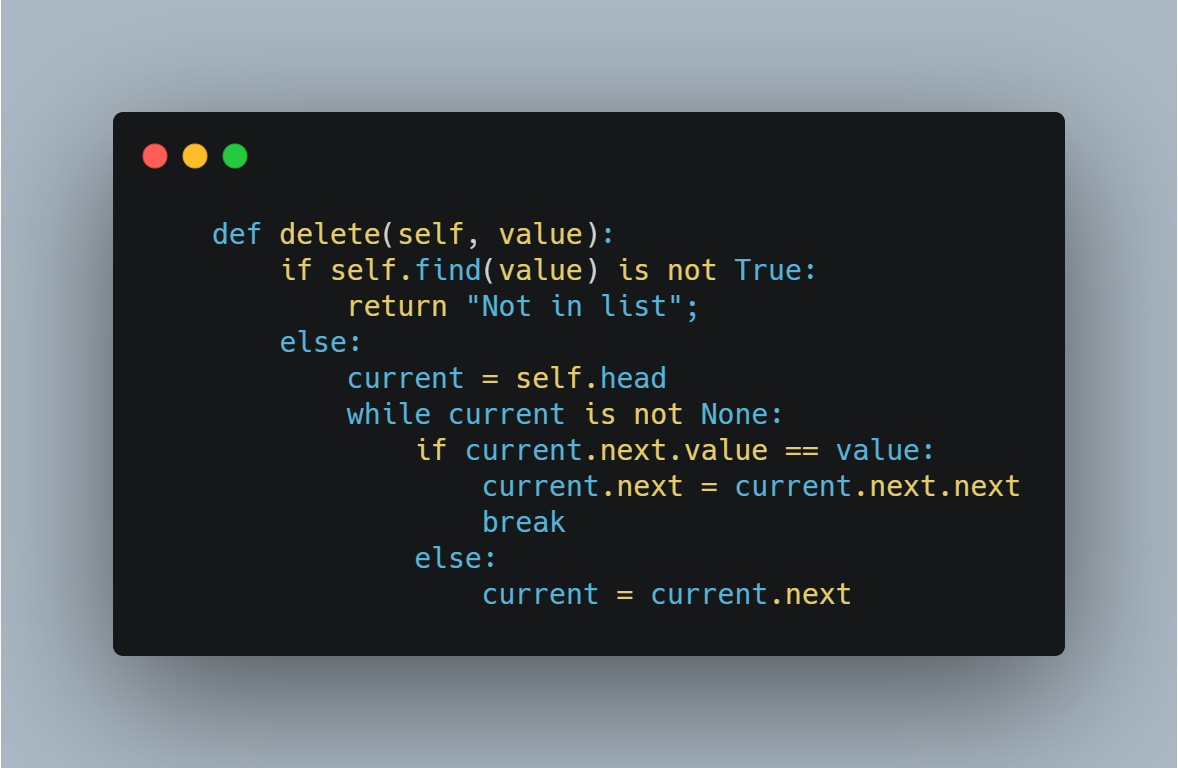Figure: Code for deleting a node in a linked list

Find element in a list

We can look for a given node in the linked list by its value. In this example, we are showing how to find element in a list.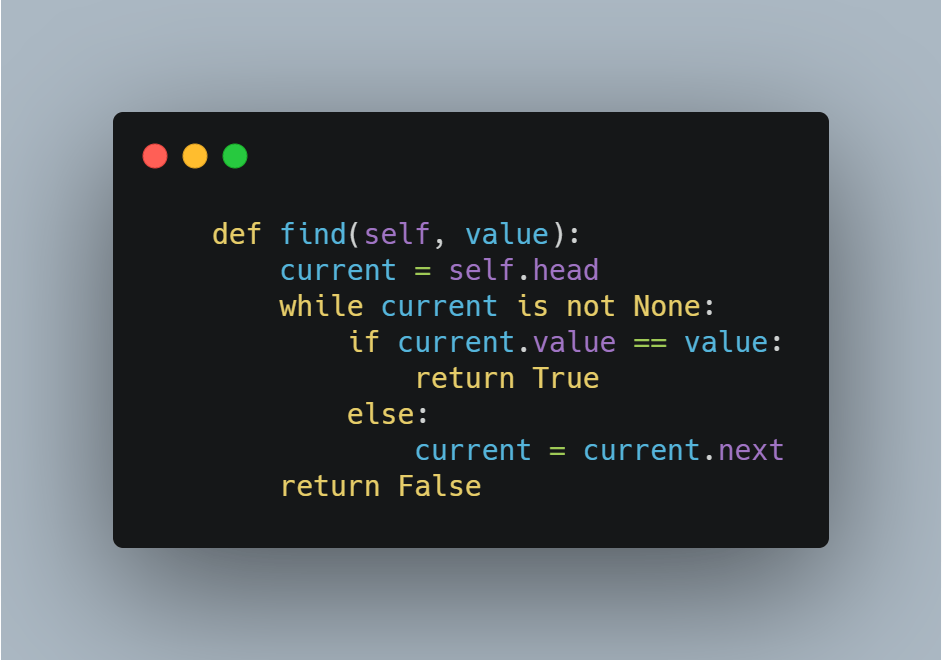Figure: Code for finding node in a linked list

We can traverse a linked list from head to tail, moving over each item. In the example shown below, we are traversing and printing value of each node.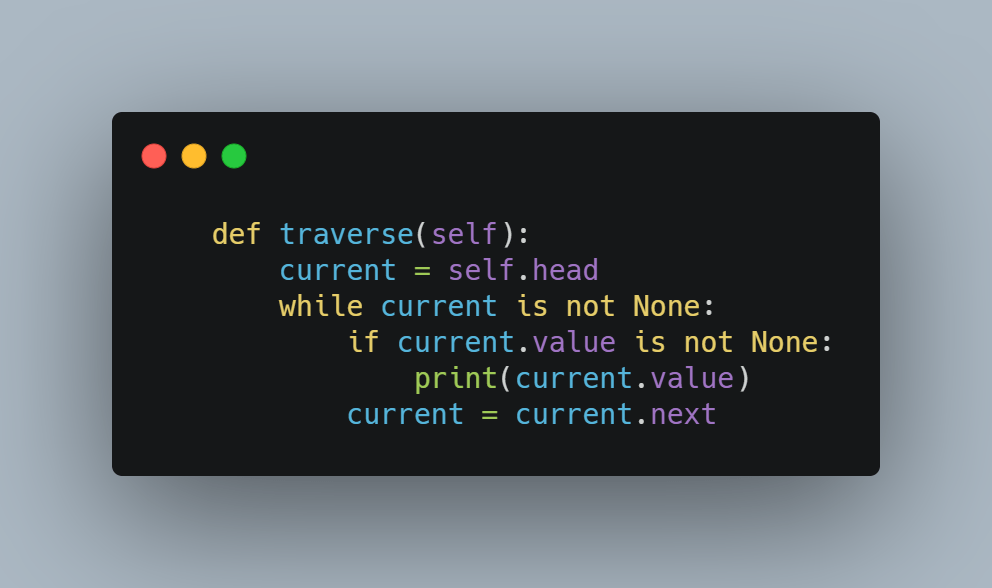Figure: Code for traversing a linked list

Linked List is one of the favorite topics of interviewers in leading tech companies like Google, Amazon, and Microsoft etc.

• How to add an element in the middle of a linked list?
• How do you reverse every alternate k nodes of a Linked List?
• How to find the length of a singly linked list?
• How do you merge two sorted lists into a single sorted linked list?
• Insert given node into the correct sorted position in the given sorted linked list
• Given a linked list, change it to be in sorted order
• Split the nodes of the given linked list into front and back halves
• How to remove duplicates from a sorted linked list
• How to detect loop in linked list
• How to remove loop in linked list
• Find nth node from the end of linked list
• Function to check if a singly linked list is a palindrome
• Find K’th node from the end in a linked list
• Merge alternate nodes of two linked lists into the first list
• Delete every N nodes in a linked list after skipping M nodes
• How to find the frequency of a given number in a Linked List?
• Write code to print out the data stored in each node in a singly linked list?
• Rearrange the linked list so that it has alternating high, low values
• Rearrange a Linked List by Separating Odd Nodes from the Even Ones
• How to flatten a linked list